Author: Oscar Cronquist Article last updated on November 12, 2019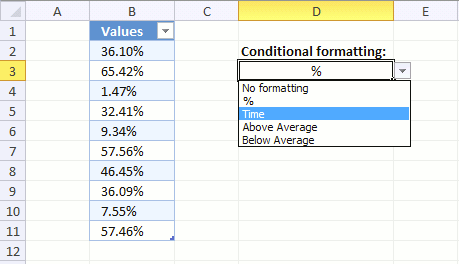This article demonstrates how to apply different cell formatting to a cell range based on a Drop Down list, column B contains an Excel Table with numerical values.

The Drop down list in cell D3 lets you choose between five different types of cell formatting, they are:

• No formatting (General)
• % (percentage)
• Numbers formatted as time
• Highlight numbers below average
• Highlight numbers above average

You can, of course, pick whatever cell formatting you want and how many or few as you want. I chose those for demonstrational purposes.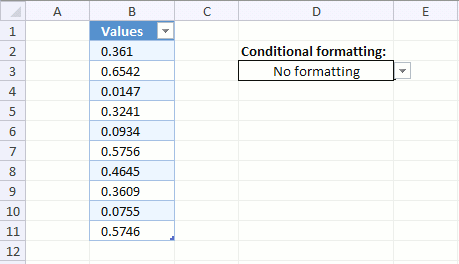The animated image above shows me selecting different formattings and column B instantly changes based on what I selected. This technique can be useful if you are building a Dashboard.

Here is how I did it.

### Create an Excel Table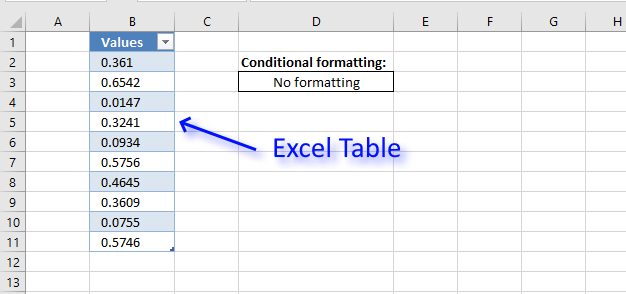This step is optional, however, I highly recommend it if you know you will be adding more values later on.

An Excel Table applies Conditional Formatting to new values automatically, this makes it really useful because there is no need to extend CF formatting or change CF formulas when new values are added.

1. Select all cells in your data set.
2. Go to tab "Insert" on the ribbon.
3. Press with left mouse button on "Table" button, a dialog box appears.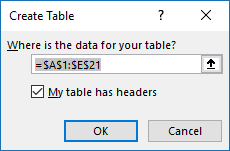4. Press with left mouse button on OK button.

You data set has now a different cell formatting applied, this is done every time data is converted to an Excel Table. You can change this if you like.

1. Press with left mouse button on any cell in your Excel Table.
2. Go to tab "Table Design" on the ribbon.
3. Here you have plenty of Table Styles to choose from.

### Create a Drop Down list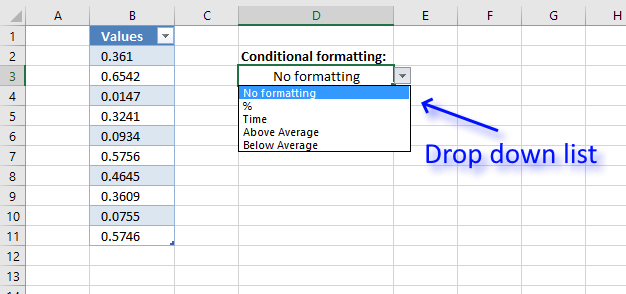A Drop Down list lets you control what the user enters in a worksheet, press with left mouse button on the black arrow next to the cell to expand the list.

The list shows valid values the user can select, simply press with left mouse button on a value with the mouse or use up/down arrow keys.

1. Select cell D3.
2. Go to tab "Data" on the ribbon.
3. Press with left mouse button on "Data Validation" button on the ribbon and a dialog box appears.
4. Go to tab "Settings" on the dialog box. See image below.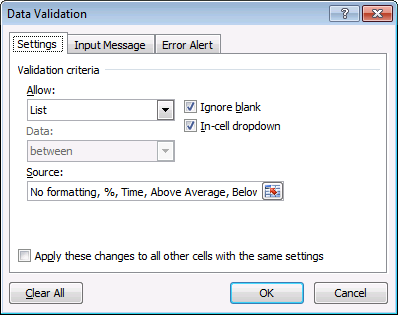5. Select List
6. Type: No formatting, %, Time, Above Average, Below Average
7. Press with left mouse button on OK button to close the dialog box.

### Apply Conditional formatting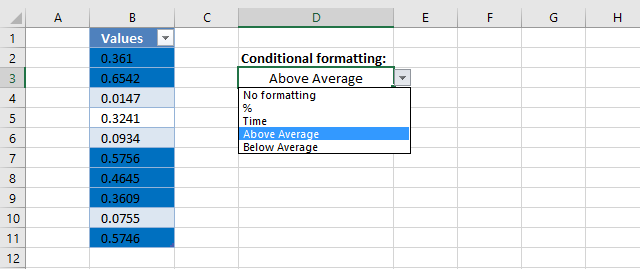Conditional Formatting allows you to format a cell or cell range based on a condition, in this case, the condition is given in cell D3 where we have a Drop Down list located.

We need to create five different CF formulas, each one applying different cell formatting to a column in the Excel Table.

For example, the image above shows that the selected value in the Drop Down list is "Above Average", the corresponding Conditional Formatting formula is activated and highlights values in Excel Table if a number is above the average of all numbers in the "Values" column.

Follow these steps to apply Conditional Formatting to column Values in the Excel Table.

1. Select all values in column "Values".
2. Go to tab "Home" on the ribbon.
3. Press with left mouse button on the "Conditional Formatting" button and a menu appears.
4. Press with left mouse button on "New Rule...".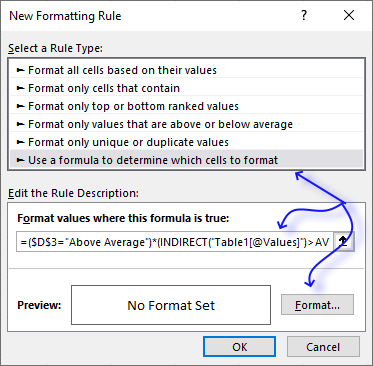5. Copy this formula:
=(\$D\$3="Above Average")*(INDIRECT("Table1[@Values]")>AVERAGE(INDIRECT("Table1[Values]")))
and paste to "Format values where this is true:"
6. Press with left mouse button on "Format..." button and pick a color. Cells that meet the requirement (return TRUE) in the above formula will be highlighted with this color.
7. Press with left mouse button on OK button.
8. Press with left mouse button on OK button once again to close the Conditional Formatting dialog box.
9. Repeat step 1 to 8 with the remaining cell formattings.

Here are the formulas and formatting:

% formula

=\$D\$3="%"

The formula checks if the value in cell D3 is %. If true the following formatting is applied: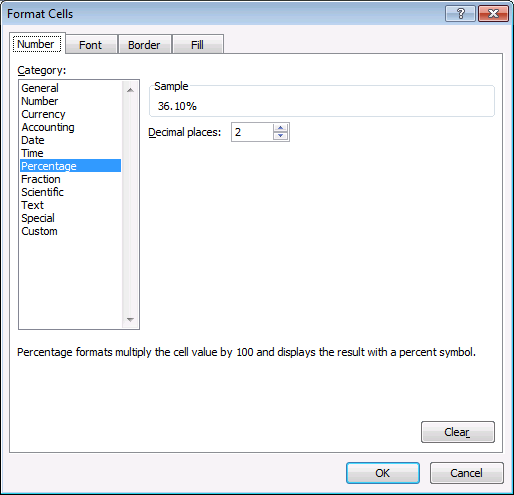Time formula

=\$D\$3="Time"

The formula checks if the value in cell D3 is Time. If true the following formatting is applied: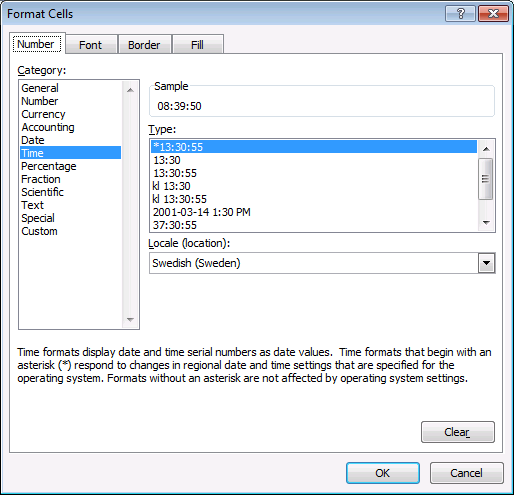Above Average

=(\$D\$3="Above Average")*(INDIRECT("Table1[@Values]")>AVERAGE(INDIRECT("Table1[Values]")))

In this formula, cell D3 is "Above Average" AND checks if each value is above the average of the table values. If TRUE the cell is highlighted.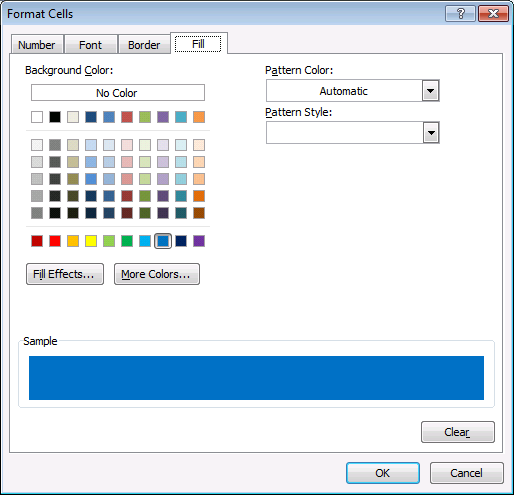Below Average

=(\$D\$3="Below Average")*(INDIRECT("Table1[@Values]")<AVERAGE(INDIRECT("Table1[Values]")))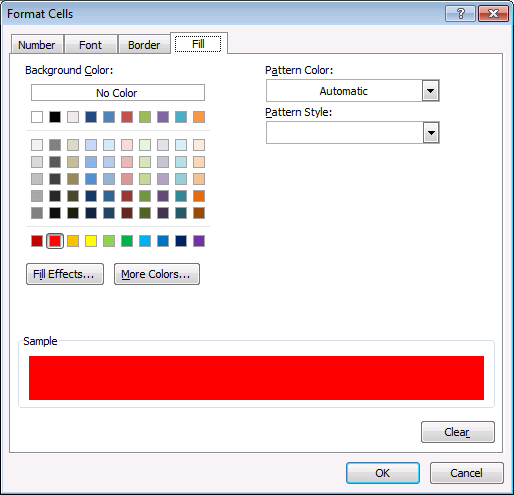### Get the Excel fileUse-a-drop-down-list-to-change-conditional-formatting.xlsx